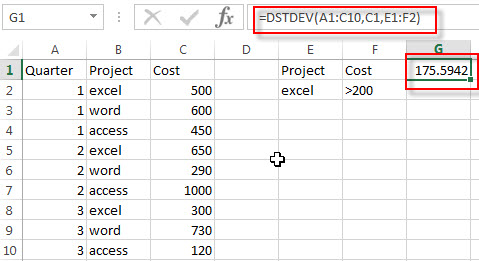## Excel DSTDEV Function

This post will guide you how to use Excel DSTDEV function with syntax and examples in Microsoft excel.

### Description

The Excel DSTDEV returns the standard deviation of a population based on a sample of numbers in a column or database that match the given criteria.

The DSTDEV function is a build-in function in Microsoft Excel and it is categorized as a Database Function.
The DSTDEV function is available in Excel 2016, Excel 2013, Excel 2010, Excel 2007, Excel 2003, Excel XP, Excel 2000, Excel 2011 for Mac.

### Syntax

The syntax of the DSTDEV function is as below:

`= DSTDEV (database, field, criteria)`

Where the DSTDEV function arguments are:

• Database -This is a required argument. The range of cells that containing the database.
• Field – This is a required argument. The column with database that you want the minimum of.
• Criteria – The range of cells that contains the conditions that you specify.

### Excel DSTDEV Function Examples

The below examples will show you how to use Excel DSTDEV Function to calculate the sample standard deviation of a column in a database or list that matching the given criteria you specify.

#1 =DSTDEV(A1:C10,C1,E1:F2)### Related Functions

• Excel DSTDEVP Function
The Excel DSTDEVP returns the standard deviation of a population based on the entire population of numbers in a column or database that match the given criteria.The syntax of the DSTDEVP function is as below:=DSTDEVP(database, field, criteria)…